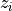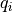# control.flatsys.FlatSystem¶

class control.flatsys.FlatSystem(forward, reverse, updfcn=None, outfcn=None, inputs=None, outputs=None, states=None, params=None, dt=None, name=None)[source]

Base class for representing a differentially flat system.

The FlatSystem class is used as a base class to describe differentially flat systems for trajectory generation. The output of the system does not need to be the differentially flat output.

Parameters
• forward (callable) – A function to compute the flat flag given the states and input.

• reverse (callable) – A function to compute the states and input given the flat flag.

• updfcn (callable, optional) –

Function returning the state update function

updfcn(t, x, u[, param]) -> array

where x is a 1-D array with shape (nstates,), u is a 1-D array with shape (ninputs,), t is a float representing the currrent time, and param is an optional dict containing the values of parameters used by the function. If not specified, the state space update will be computed using the flat system coordinates.

• outfcn (callable) –

Function returning the output at the given state

outfcn(t, x, u[, param]) -> array

where the arguments are the same as for upfcn. If not specified, the output will be the flat outputs.

• inputs (int, list of str, or None) – Description of the system inputs. This can be given as an integer count or as a list of strings that name the individual signals. If an integer count is specified, the names of the signal will be of the form s[i] (where s is one of u, y, or x). If this parameter is not given or given as None, the relevant quantity will be determined when possible based on other information provided to functions using the system.

• outputs (int, list of str, or None) – Description of the system outputs. Same format as inputs.

• states (int, list of str, or None) – Description of the system states. Same format as inputs.

• dt (None, True or float, optional) – System timebase. None (default) indicates continuous time, True indicates discrete time with undefined sampling time, positive number is discrete time with specified sampling time.

• params (dict, optional) – Parameter values for the systems. Passed to the evaluation functions for the system as default values, overriding internal defaults.

• name (string, optional) – System name (used for specifying signals)

Notes

The class must implement two functions:

zflag = flatsys.foward(x, u, params)

This function computes the flag (derivatives) of the flat output. The inputs to this function are the state ‘x’ and inputs ‘u’ (both 1D arrays). The output should be a 2D array with the first dimension equal to the number of system inputs and the second dimension of the length required to represent the full system dynamics (typically the number of states)

x, u = flatsys.reverse(zflag, params)

This function system state and inputs give the the flag (derivatives) of the flat output. The input to this function is an 2D array whose first dimension is equal to the number of system inputs and whose second dimension is of length required to represent the full system dynamics (typically the number of states). The output is the state x and inputs u (both 1D arrays).

A flat system is also an input/output system supporting simulation, composition, and linearization. If the update and output methods are given, they are used in place of the flat coordinates.

Methods

 `copy` Make a copy of an input/output system `dynamics` Compute the dynamics of a differential or difference equation. `feedback` Feedback interconnection between two input/output systems `find_input` Find the index for an input given its name (None if not found) `find_output` Find the index for an output given its name (None if not found) `find_state` Find the index for a state given its name (None if not found) `forward` Compute the flat flag given the states and input. `isctime` Check to see if a system is a continuous-time system `isdtime` Check to see if a system is a discrete-time system `issiso` Check to see if a system is single input, single output `linearize` Linearize an input/output system at a given state and input. `output` Compute the output of the system `reverse` Compute the states and input given the flat flag. `set_inputs` Set the number/names of the system inputs. `set_outputs` Set the number/names of the system outputs. `set_states` Set the number/names of the system states.

Add two input/output systems (parallel interconnection)

__call__(u, params=None, squeeze=None)[source]

Evaluate a (static) nonlinearity at a given input value

If a nonlinear I/O system has no internal state, then evaluating the system at an input u gives the output y = F(u), determined by the output function.

Parameters
• params (dict, optional) – Parameter values for the system. Passed to the evaluation function for the system as default values, overriding internal defaults.

• squeeze (bool, optional) – If True and if the system has a single output, return the system output as a 1D array rather than a 2D array. If False, return the system output as a 2D array even if the system is SISO. Default value set by config.defaults[‘control.squeeze_time_response’].

__mul__(sys1)[source]

Multiply two input/output systems (series interconnection)

__neg__()[source]

Negate an input/output systems (rescale)

Parallel addition of input/output system to a compatible object.

__rmul__(sys2)[source]

Pre-multiply an input/output systems by a scalar/matrix

__rsub__(sys2)[source]

Parallel subtraction of I/O system to a compatible object.

__sub__(sys2)[source]

Subtract two input/output systems (parallel interconnection)

__truediv__(sys1)[source]

Division of input/output systems

Only division by scalars and arrays of scalars is supported

copy(name=None, use_prefix_suffix=True)[source]

Make a copy of an input/output system

A copy of the system is made, with a new name. The name keyword can be used to specify a specific name for the system. If no name is given and use_prefix_suffix is True, the name is constructed by prepending config.defaults[‘namedio.duplicate_system_name_prefix’] and appending config.defaults[‘namedio.duplicate_system_name_suffix’]. Otherwise, a generic system name of the form sys[<id>] is used, where <id> is based on an internal counter.

dynamics(t, x, u, params=None)[source]

Compute the dynamics of a differential or difference equation.

Given time t, input u and state x, returns the value of the right hand side of the dynamical system. If the system is continuous, returns the time derivative

dx/dt = f(t, x, u[, params])

where f is the system’s (possibly nonlinear) dynamics function. If the system is discrete-time, returns the next value of x:

x[t+dt] = f(t, x[t], u[t][, params])

where t is a scalar.

The inputs x and u must be of the correct length. The params argument is an optional dictionary of parameter values.

Parameters
• t (float) – the time at which to evaluate

• x (array_like) – current state

• u (array_like) – input

• params (dict (optional)) – system parameter values

Returns

dx/dt or x[t+dt]

Return type

ndarray

feedback(other=1, sign=-1, params=None)[source]

Feedback interconnection between two input/output systems

Parameters
• sys1 (InputOutputSystem) – The primary process.

• sys2 (InputOutputSystem) – The feedback process (often a feedback controller).

• sign (scalar, optional) – The sign of feedback. sign = -1 indicates negative feedback, and sign = 1 indicates positive feedback. sign is an optional argument; it assumes a value of -1 if not specified.

Returns

out

Return type

InputOutputSystem

Raises

ValueError – if the inputs, outputs, or timebases of the systems are incompatible.

find_input(name)[source]

Find the index for an input given its name (None if not found)

find_output(name)[source]

Find the index for an output given its name (None if not found)

find_state(name)[source]

Find the index for a state given its name (None if not found)

forward(x, u, params=None)[source]

Compute the flat flag given the states and input.

Given the states and inputs for a system, compute the flat outputs and their derivatives (the flat “flag”) for the system.

Parameters
• x (list or array) – The state of the system.

• u (list or array) – The input to the system.

• params (dict, optional) – Parameter values for the system. Passed to the evaluation functions for the system as default values, overriding internal defaults.

Returns

zflag – For each flat output, zflag[i] should be an ndarray of lengththat contains the flat output and its firstderivatives.

Return type

list of 1D arrays

isctime(strict=False)[source]

Check to see if a system is a continuous-time system

Parameters
• sys (Named I/O system) – System to be checked

• strict (bool, optional) – If strict is True, make sure that timebase is not None. Default is False.

isdtime(strict=False)[source]

Check to see if a system is a discrete-time system

Parameters

strict (bool, optional) – If strict is True, make sure that timebase is not None. Default is False.

issiso()[source]

Check to see if a system is single input, single output

linearize(x0, u0, t=0, params=None, eps=1e-06, name=None, copy_names=False, **kwargs)[source]

Linearize an input/output system at a given state and input.

Return the linearization of an input/output system at a given state and input value as a StateSpace system. See `linearize()` for complete documentation.

ninputs

Number of system inputs.

noutputs

Number of system outputs.

nstates

Number of system states.

output(t, x, u, params=None)[source]

Compute the output of the system

Given time t, input u and state x, returns the output of the system:

y = g(t, x, u[, params])

The inputs x and u must be of the correct length.

Parameters
• t (float) – the time at which to evaluate

• x (array_like) – current state

• u (array_like) – input

• params (dict (optional)) – system parameter values

Returns

y

Return type

ndarray

reverse(zflag, params=None)[source]

Compute the states and input given the flat flag.

Parameters
• zflag (list of arrays) – For each flat output, zflag[i] should be an ndarray of lengththat contains the flat output and its firstderivatives.

• params (dict, optional) – Parameter values for the system. Passed to the evaluation functions for the system as default values, overriding internal defaults.

Returns

• x (1D array) – The state of the system corresponding to the flat flag.

• u (1D array) – The input to the system corresponding to the flat flag.

set_inputs(inputs, prefix='u')[source]

Set the number/names of the system inputs.

Parameters
• inputs (int, list of str, or None) – Description of the system inputs. This can be given as an integer count or as a list of strings that name the individual signals. If an integer count is specified, the names of the signal will be of the form u[i] (where the prefix u can be changed using the optional prefix parameter).

• prefix (string, optional) – If inputs is an integer, create the names of the states using the given prefix (default = ‘u’). The names of the input will be of the form prefix[i].

set_outputs(outputs, prefix='y')[source]

Set the number/names of the system outputs.

Parameters
• outputs (int, list of str, or None) – Description of the system outputs. This can be given as an integer count or as a list of strings that name the individual signals. If an integer count is specified, the names of the signal will be of the form u[i] (where the prefix u can be changed using the optional prefix parameter).

• prefix (string, optional) – If outputs is an integer, create the names of the states using the given prefix (default = ‘y’). The names of the input will be of the form prefix[i].

set_states(states, prefix='x')[source]

Set the number/names of the system states.

Parameters
• states (int, list of str, or None) – Description of the system states. This can be given as an integer count or as a list of strings that name the individual signals. If an integer count is specified, the names of the signal will be of the form u[i] (where the prefix u can be changed using the optional prefix parameter).

• prefix (string, optional) – If states is an integer, create the names of the states using the given prefix (default = ‘x’). The names of the input will be of the form prefix[i].